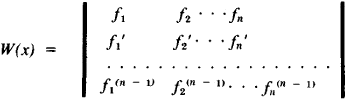# Wronskian

Also found in: Wikipedia.

## Wronskian

[′vrän·skē·ən]
(mathematics)
An n × n matrix whose i th row is a list of the (i - 1)st derivatives of a set of functions f1, …, fn ; ordinarily used to determine linear independence of solutions of linear homogeneous differential equations.

## Wronskian

a functional determinant composed of n functions f1(x), f2(x)....,fn(x) and their derivatives up to the order n - 1 inclusive:The vanishment of the Wrońskian [W(x) = 0] is a necessary and, under certain additional assumptions, a sufficient condition for the linear dependence between the given n functions, differentiated n - 1 times. Based on this, the Wrońskian is used in the theory of linear differential equations. The Wrońskian was introduced by J. Wroński in 1812.

References in periodicals archive ?
Thus a dual Grothendieck polynomial is still a discrete Wronskian that one identifies with a multiSchur function (in the case of an increasing or decreasing sequence of alphabets, one also uses the term flagged Schur function cf.
where <y, z> := yz' - y'z is the Wronskian of y and z.
If X, Y [member of] D, then we define the Wronskian matrix of X(t) and Y(t) by
21) ensures that the Wronskian matrix of the linearly independent functions ([v.
are linearly independent by computing its Wronskian at t = 0.
W is the Wronskian of the two independent solutions, which is constant, being the layer matrix M unimodular, and:
4) are linearly independent if and only if their Wronskian is different from zero.
i] are linearly independent over F, then there exists a generalized Wronskian of the (3), which does not vanish.
The accuracy of the results can be checked using the Wronskians for Mathieu functions, as the algorithms for computing Mathieu functions are not based on the Wronskian of the functions.
Since the Wronskian of any two solution of (8) is independent of t, while t [right arrow] [infinity] in (11) we also get
Marini, On intermediate solutions and the Wronskian for half-linear differential equations, J.
This generalization is obtained by considering the Wronskian of functions over F[x].

Site: Follow: Share:
Open / Close